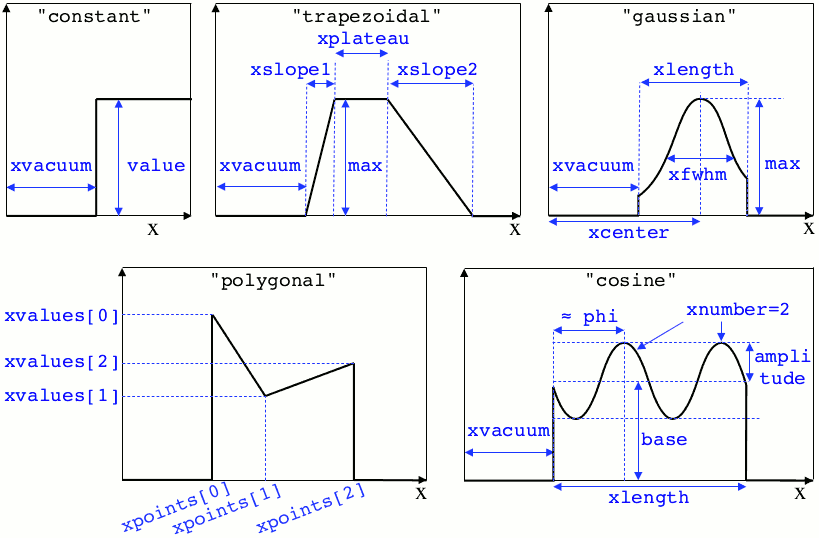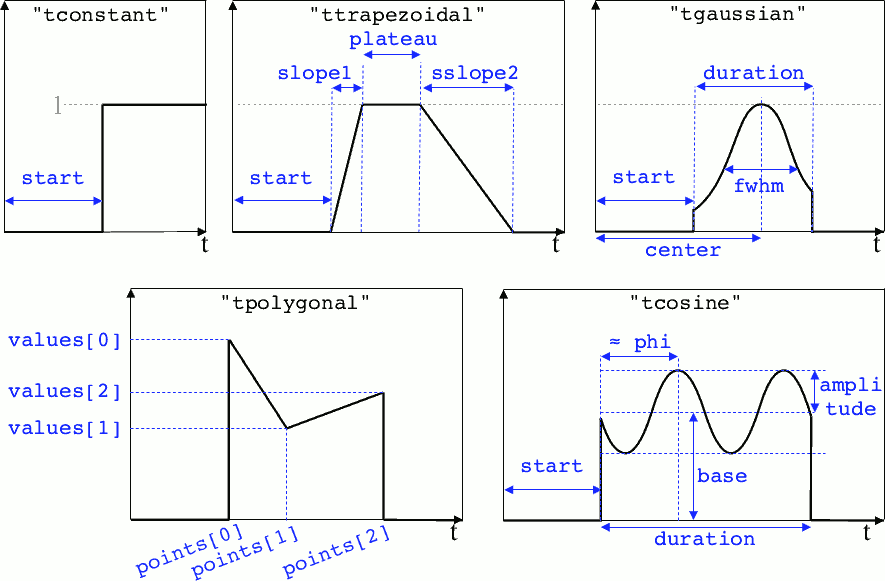# Profiles¶

Several quantities require the input of a profile: particle charge, particle density, external fields, etc. Depending on the case, they can be spatial or temporal profiles.

## Constant profiles¶

• Species( ... , charge = -3., ... ) defines a species with charge $$Z^\star=3$$.

• Species( ... , number_density = 10., ... ) defines a species with density $$10\,N_r$$. You can choose number_density or charge_density

• Species( ... , mean_velocity = [0.05, 0., 0.], ... ) defines a species with drift velocity $$v_x = 0.05\,c$$ over the whole box.

• Species(..., momentum_initialization="maxwell-juettner", temperature=[1e-5], ...) defines a species with a Maxwell-Jüttner distribution of temperature $$T = 10^{-5}\,m_ec^2$$ over the whole box. Note that the temperature may be anisotropic: temperature=[1e-5, 2e-5, 2e-5].

• Species( ... , particles_per_cell = 10., ... ) defines a species with 10 particles per cell.

• ExternalField( field="Bx", profile=0.1 ) defines a constant external field $$B_x = 0.1 B_r$$.

## Python profiles¶

Any python function can be a profile. Examples:

def f(x):
if x<1.: return 0.
else: return 1.

import math
def f(x,y):    # two variables for 2D simulation
twoPI = 2.* math.pi
return math.cos(  twoPI * x/3.2 )

f = lambda x: x**2 - 1.


Once the function is created, you have to include it in the block you want, for example:

Species( ... , charge = f, ... )

Species( ... , mean_velocity = [f, 0, 0], ... )


Note

It is possible, for higher performances, to create functions with arguments (x, y, etc.) that are actually numpy arrays. If the function returns a numpy array of the same size, it will automatically be considered as a profile acting on arrays instead of single floats. Currently, this feature is only available on Species’ profiles.

## Pre-defined spatial profiles¶

constant(value, xvacuum=0., yvacuum=0.)
Parameters
• value – the magnitude

• xvacuum – vacuum region before the start of the profile.

trapezoidal(max, xvacuum=0., xplateau=None, xslope1=0., xslope2=0., yvacuum=0., yplateau=None, yslope1=0., yslope2=0.)
Parameters
• max – maximum value

• xvacuum – empty length before the ramp up

• xplateau – length of the plateau (default is grid_length $$-$$ xvacuum)

• xslope1 – length of the ramp up

• xslope2 – length of the ramp down

gaussian(max, xvacuum=0., xlength=None, xfwhm=None, xcenter=None, xorder=2, yvacuum=0., ylength=None, yfwhm=None, ycenter=None, yorder=2)
Parameters
• max – maximum value

• xvacuum – empty length before starting the profile

• xlength – length of the profile (default is grid_length $$-$$ xvacuum)

• xfwhm – gaussian FWHM (default is xlength/3.)

• xcenter – gaussian center position (default is in the middle of xlength)

• xorder – order of the gaussian.

Note

If yorder equals 0, then the profile is constant over $$y$$.

polygonal(xpoints=[], xvalues=[])
Parameters
• xpoints – list of the positions of the points

• xvalues – list of the values of the profile at each point

cosine(base, amplitude=1., xvacuum=0., xlength=None, xphi=0., xnumber=1)
Parameters
• base – offset of the profile value

• amplitude – amplitude of the cosine

• xvacuum – empty length before starting the profile

• xlength – length of the profile (default is grid_length $$-$$ xvacuum)

• xphi – phase offset

• xnumber – number of periods within xlength

polynomial(x0=0., y0=0., z0=0., order0=[], order1=[], ...)
Parameters
• x0,y0 – The reference position(s)

• order0 – Coefficient for the 0th order

• order1 – Coefficient for the 1st order (2 coefficients in 2D)

• order2 – Coefficient for the 2nd order (3 coefficients in 2D)

• etc

Creates a polynomial of the form

$\begin{split}\begin{eqnarray} &\sum_i a_i(x-x_0)^i & \quad\mathrm{in\, 1D}\\ &\sum_i \sum_j a_{ij}(x-x0)^{i-j}(y-y0)^j & \quad\mathrm{in\, 2D}\\ &\sum_i \sum_j \sum_k a_{ijk}(x-x0)^{i-j-k}(y-y0)^j(z-z0)^k & \quad\mathrm{in\, 3D} \end{eqnarray}\end{split}$

Each orderi is a coefficient (or list of coefficents) associated to the order i. In 1D, there is only one coefficient per order. In 2D, each orderi is a list of i+1 coefficients. For instance, the second order has three coefficients associated to $$x^2$$, $$xy$$ and $$y^2$$, respectively. In 3D, each orderi is a list of (i+1)*(i+2)/2 coefficients. For instance, the second order has 6 coefficients associated to $$x^2$$, $$xy$$, $$xz$$, $$y^2$$, $$yz$$ and $$z^2$$, respectively.

Examples:

Species( ... , density = gaussian(10., xfwhm=0.3, xcenter=0.8), ... )

ExternalField( ..., profile = constant(2.2), ... )


Illustrations of the pre-defined spatial profiles## Pre-defined temporal profiles¶

tconstant(start=0.)
Parameters

start – starting time

ttrapezoidal(start=0., plateau=None, slope1=0., slope2=0.)
Parameters
• start – starting time

• plateau – duration of the plateau (default is simulation_time $$-$$ start)

• slope1 – duration of the ramp up

• slope2 – duration of the ramp down

tgaussian(start=0., duration=None, fwhm=None, center=None, order=2)
Parameters
• start – starting time

• duration – duration of the profile (default is simulation_time $$-$$ start)

• fwhm – gaussian FWHM (default is duration/3.)

• center – gaussian center time (default is in the middle of duration)

• order – order of the gaussian

tpolygonal(points=[], values=[])
Parameters
• points – list of times

• values – list of the values at each time

tcosine(base=0., amplitude=1., start=0., duration=None, phi=0., freq=1.)
Parameters
• base – offset of the profile value

• amplitude – amplitude of the cosine

• start – starting time

• duration – duration of the profile (default is simulation_time $$-$$ start)

• phi – phase offset

• freq – frequency

tpolynomial(t0=0., order0=[], order1=[], ...)
Parameters
• t0 – The reference position

• order0 – Coefficient for the 0th order

• order1 – Coefficient for the 1st order

• order2 – Coefficient for the 2nd order

• etc

Creates a polynomial of the form $$\sum_i a_i(t-t_0)^i$$.

tsin2plateau(start=0., fwhm=0., plateau=None, slope1=fwhm, slope2=slope1)
Parameters
• start – Profile is 0 before start

• fwhm – Full width half maximum of the profile

• plateau – Length of the plateau

• slope1 – Duration of the ramp up of the profil

• slope2 – Duration of the ramp down of the profil

Creates a sin squared profil with a plateau in the middle if needed. If slope1 and 2 are used, fwhm is overwritten.

Example:

Antenna( ... , time_profile = tcosine(freq=0.01), ... )


Illustrations of the pre-defined temporal profiles## Extract the profile from a file¶

The following profiles may be given directly as an HDF5 file:

• Species.charge_density

• Species.number_density

• Species.particles_per_cell

• Species.charge

• Species.mean_velocity

• Species.temperature

• ExternalField.profile except when complex (cylindrical geometry)

You must provide the path to the file, and the path to the dataset inside the file. For instance charge_density = "myfile.h5/path/to/dataset".

The targeted dataset located in the file must be an array with the same dimension and the same number of cells as the simulation grid.

Warning

For ExternalField, the array size must take into account the number of ghost cells in each direction. There is also one extra cell in specific directions due to the grid staggering (see this doc).